Excel16 -6- Pivot tables

Learn to create and use pivot tables to rapidly extract key statistics from a large data set.

1. What is a pivot table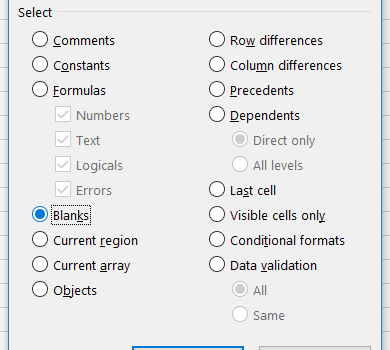Everything starts with a clean dataset! A clean dataset means that: the independent and dependent variables are organised in columns, each column has a unique title (header), each row has only one entry per variable, empty cells in the table should be avoided.   Let’s take an example. We conduct an […]

2. Preparing the table for a pivot table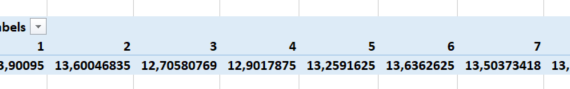To create a pivot table from the dataset introduced previously here (and downloadable here), select the table (A1-H721), choose Insert in the main menu, and Pivot Table.                 In the dialog box that shows up, you may choose the data (if it is […]

3. Creating the pivot table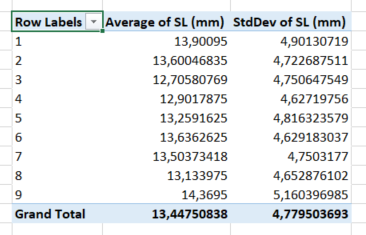In this post, we keep using the example introduced here. We had built a pivot table showing the standard length SL (mm) for each of the 9 tanks.     The side menu looked like this.     And the pivot table looked like this.               […]

4. Displaying several statistics in a pivot table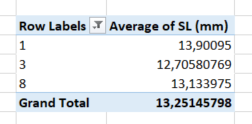In this post, we keep using the example introduced here. We had built a pivot table showing the standard length SL (mm) for each of the 9 tanks.     The side menu looked like this…     …and the pivot table looked like this.             In […]

5. Displaying a subset of a category in a pivot table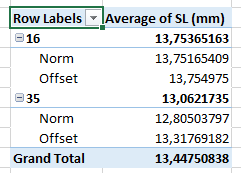In this post, we keep using the example introduced here. We had built a pivot table showing the standard length SL (mm) for each of the 9 tanks.     The side menu looked like this…     …and the pivot table looked like this.             In […]